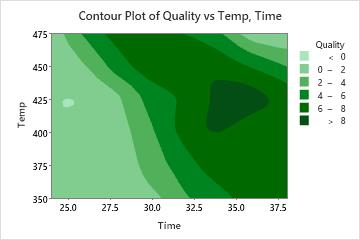# Overview for Contour Plot

Use Contour Plot to examine the relationship between a response variable and two predictor variables. In a contour plot, the values for two predictor variables are represented on the x- and y-axes, and the values for the response variable are represented by shaded regions, called contours. A contour plot is like a topographical map in which x-, y-, and z-values are plotted instead of longitude, latitude, and altitude.

For example, the following contour plot shows the effect of temperature and time on the quality of reheated frozen entrees.## Where to find this graph

To create a contour plot, choose Graph > Contour Plot.

By using this site you agree to the use of cookies for analytics and personalized content.  Read our policy